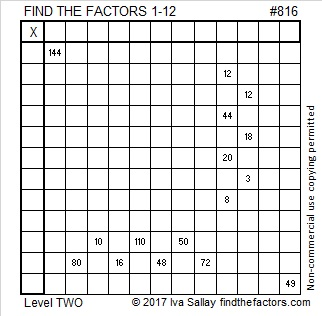# 816 and Level 2

Eight is half of sixteen, so 816 is divisible by 6. You probably weren’t expecting that divisibility rule, but it’s true.

816 can also be easily divided by 2, 4, and 8. How many factors does 816 have in all? Plenty! Scroll down past the puzzle and see!Print the puzzles or type the solution on this excel file: 12 factors 815-820

• 816 is a composite number.
• Prime factorization: 816 = 2 x 2 x 2 x 2 x 3 x 17, which can be written 816 = 2⁴ x 3 x 17
• The exponents in the prime factorization are 4, 1 and 1. Adding one to each and multiplying we get (4 + 1)(1 + 1)(1 + 1) = 5 x 2 x 2 = 20. Therefore 816 has exactly 20 factors.
• Factors of 816: 1, 2, 3, 4, 6, 8, 12, 16, 17, 24, 34, 48, 51, 68, 102, 136, 204, 272, 408, 816
• Factor pairs: 816 = 1 x 816, 2 x 408, 3 x 272, 4 x 204, 6 x 136, 8 x 102, 12 x 68, 16 x 51, 17 x 48 or 24 x 34
• Taking the factor pair with the largest square number factor, we get √816 = (√16)(√51) = 4√51 ≈ 28.5657Since 17 is one of its factors, 816 is the hypotenuse of a Pythagorean triple:

• 384-720-816 which is 48 times 8-15-17

816 is repdigit OO in base 33 (O is 24 base 10). That is true because

• 24(33¹) + 24(33º) = 24(33¹ + 33º) = 24(33 + 1) = 24 × 34 = 816

816 is the sum of the sixteen prime numbers from 19 to 83:

• 19 + 23 + 29 + 31 + 37 + 41 + 43 + 47 + 53 + 59 + 61 + 67 + 71 + 73 + 79 + 83 = 816

Coincidentally, 816 is also the sixteenth tetrahedral number.

That’s because 16(16 + 1)(16 + 2)/6 = 816, which is a fast way to compute it. Here’s what it means to be the 16th tetrahedral number:This site uses Akismet to reduce spam. Learn how your comment data is processed.